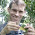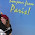## Thursday, November 29, 2012

### Issue with plotting output trees from SIMMAP using densityMap

A phytools user contacted me yesterday with the following issue:

I was trying to use your densityMap function to visualize some output from SIMMAP. The mutational mappings seemed to work fine in SIMMAP. I tried importing into R using read.simmap, this seemed to work fine. I then tried to use densityMap but it keeps giving me the following error:
> densityMap(trees,res=1000)
sorry - this might take a while; please be patient
Error in lower:upper: NA/NaN argument

This isn't something that I'd seen before, but I recognized that the code is from a part of the function in which the beginning and ending points for each map segment on a branch (XX) is compared to a matrix containing the beginning and ending points for every step interval along the same edge (YY); in which these steps are based on the edge length of the branch and the function argument res. Importantly, the beginnings and endings are in terms of the height above the root.

This made me suspect that the problem was with the edge lengths of the tree. densityMap assumes that the topology of the input trees are equal with proportional branch lengths. (In fact, there is an option - densityMap(...,check=TRUE) - that evaluates this equality criterion, and returns an error message if it fails.) For some reason that I don't completely understand (and even when 'mutational mapping' is performed on a single input tree), SIMMAP returns a sample of trees with varying total length. Theoretically, this should not be a problem if the branch lengths are strictly proportional. Unfortunately, due to numerical precision (which is relatively low - 6 digit - in the SIMMAP output), the branch lengths of these varyingly scaled trees are not strictly proportional. Consequently, even after they have been scaled to the same total length using phytools function rescaleSimmap (which is done internally in densityMap), the trees have slightly different branch lengths - sufficiently different, evidently, to account for this error.

My eventual workaround was to write a function that would apply one set of branch lengths (say, from a reference tree) to another tree or set of trees. For basic "phylo" objects, this is trivial of course. We would just do:
# for reference tree tr1
tr2\$edge.length<-tr1\$edge.length

However, for trees with mappings, it is slightly more complicated. Here is a simplified version of the function:
applyBranchLengths<-function(tree,edge.length){
if(class(tree)=="multiPhylo"){
trees<-lapply(tree,applyBranchLengths,
edge.length=edge.length)
class(trees)<-"multiPhylo"
return(trees)
} else {
tree\$edge.length<-edge.length
if(!is.null(tree\$maps)){
for(i in 1:nrow(tree\$edge)){
temp<-tree\$maps[[i]]/sum(tree\$maps[[i]])
tree\$maps[[i]]<-temp*tree\$edge.length[i]
}
}
return(tree)
}
}

This function uses the proportional time spent in each state along each edge of the target tree in rescaling the mappings to the reference tree. (I've left out calculation of the \$mapped.edge matrix here, which involves a few more lines of code.) I have posted the full function code for this here.

Anyway, it worked! Here's a quick demo with a simulated tree & dataset in which I performed stochastic mapping using SIMMAP v1.5 for another project:
> densityMap(trees)
sorry - this might take a while; please be patient
Error in lower:upper : NA/NaN argument
> # get the tree heights
> h<-sapply(trees,function(x) max(nodeHeights(x)))
> h
 13.215321  6.213479 16.864350  9.936482 ....
...
> # ok, they have different heights - is that the problem?
> # try rescaling
> rescaled<-rescaleSimmap(trees,mean(h))
> densityMap(rescaled)
sorry - this might take a while; please be patient
Error in lower:upper : NA/NaN argument
> # nope!
> # ok, how about *not* rescaling, but applying the
> # relative branch lengths from trees[] to all the
> # trees in the sample
> newbl<-mapply(applyBranchLengths,trees, lapply(as.list(h),"*",trees[]\$edge.length/h), SIMPLIFY=FALSE)
> class(newbl)<-"multiPhylo"
> # these trees have their original total lengths
> # but relative branch lengths from trees[]
> densityMap(newbl,fsize=c(0.6,1))
sorry - this might take a while; please be patient

1.I forgot to note that when the total lengths of the sample of stochastic mapped trees vary, densityMap presently (arbitrarily) plots a tree with a total length equal to the longest tree in the sample. This easily be changed to use the mean or a user specified total length.

2.Hi Liam,

I'm attempting to summarize SIMMAP v1.5 mutational mappings in densityMap. I receive the same error "Error in lower:upper : NA/NaN argument" reported here. This continues even after performing the fix you outline above. The edge.lengths of the trees are identical after this correction, but I still get the same error. Do you have any suggestions?

Thanks for your help!

Mandy

1.Hi Mandy.

Do you have any zero length internal branches in your tree? This could also be the explanation. Try any(tree\$edge.length==0). Very short internal branches can also cause a problem.

I will shortly post a solution to this based on a new function that collapses branches of zero length into polytomies for stochastically mapped trees.

If that is not your problem - it would be very helpful if you send me your saved workspace (.Rdata file) so that I can investigate this issue further.

Thanks! Liam

2.Mandy. This might be of use. Please let me know if you need a Windows or Mac OS build of phytools to install the latest version & try it out on your dataset. - Liam

3.Hi Liam,

Thank you very much for your response! There are zero and small branch lengths in my tree, so this issue is a good candidate. Unfortunately, I am still encountering the same error with the new fix. I will email you my .Rdata file.

Thanks again,
Mandy

1.Hi Mandy.

Response coming by email. In short, your input file has only 2 trees from the posterior distribution. You need (say) 100 or 1,000 sampled trees in order to use densityMap. I'll shortly email you what I did & the results.

- Liam

4.This comment has been removed by the author.

Note: due to the very large amount of spam, all comments are now automatically submitted for moderation.